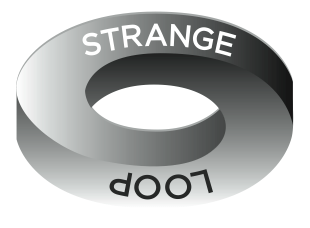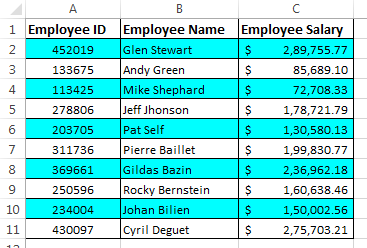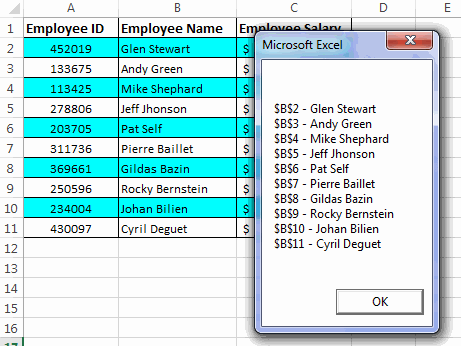# VBA Loops – For, For Each, Do While and Do Until Loops

Loops are used in VBA for repeating a set of statements multiple times. Loops form a very important part of any programming language and VBA is no exception. There are different types of loops that can be used in VBA. These are as follows:

1. VBA For Loop
2. VBA For Each Loop
3. VBA Do While Loop
4. VBA Do Until Loop

In this post I will explain all these VBA Loops with examples.### What is a loop and what are its uses?

As I have foretold that a loop is an instruction that has the ability to continually repeat a set of statements until a certain condition is reached.

The basic purpose of loops are as follows:

• It helps in iterating a set of statements.
• It helps in checking a particular condition multiple times.
• It can also help in developing sleep and wait logics in code.

### VBA For Loop:

For loop is one of the most important and frequently used loop in VBA. For Loop is sometimes also called as For Next Loop.

The basic syntax of For loop is as follows:

`For loop_ctr = start_num To end_num 'Statements to be executed inside the loopNext loop_ctr`

Here, ‘`loop_ctr`’ stands for loop counter. It is the backbone of the loop and hence it is also called ‘`loop time keeper`’. This variable gets incremented after each iteration until the loop ends.

`start_num`’ is the number from which the loop should begin.

`end_num`’ is the number till which the loop should continue.

How a For Loop works:

Let’s say we have a simple For Loop as shown below:

`For loop_ctr = 1 To 100 'Statements to be executed inside the loopNext loop_ctr`
• When the program control reaches the statement `For loop_ctr = 1 To 100`, it reserves a space for the variable ‘`loop_ctr`’ in the memory and initializes it to 1.
• After this, it executes the statements inside the For loop sequentially.
• Finally the program control reaches the statement `Next loop_ctr`, here it increments the variable ‘`loop_ctr`’ by 1. And then again goes to the statement `For loop_ctr = 1 To 100` here it checks if the value of ‘`loop_ctr`’ has reached 100 or not. If the value is less than 100 then it continues next iteration otherwise the loop stops.

Few practical examples of VBA For Loop:

Now let’s have a look at some of the practical examples where For Loop can be used:

Example 1: In this example we have a range “A1:A100” and we have to fill this range with numbers from 1-100.To accomplish this we can use the below code:

`Sub ForLoopTest()Dim loop_ctr As IntegerFor loop_ctr = 1 To 100 ActiveSheet.Range("A" & loop_ctr).Value = loop_ctrNext loop_ctrMsgBox "For Loop Completed!"End Sub`

Explanation:

In the above code the loop runs for 100 times and populates the cells in the range A1:A100 with numbers from 1-100 serially.

Note: Here we have used the MsgBox for telling the user that the loop has executed.

Example 2: In this example we have a table on a sheet and now we need to highlight alternate rows in the table.To do this we can use the below code:

`Sub ForLoopTest()Dim loop_ctr As IntegerDim Max As IntegerDim clm As IntegerMax = ActiveSheet.UsedRange.Rows.Countclm = ActiveSheet.UsedRange.Columns.CountFor loop_ctr = 1 To Max If loop_ctr Mod 2 = 0 Then ActiveSheet.Range(Cells(loop_ctr, 1), Cells(loop_ctr, clm)).Interior.ColorIndex = 28 End IfNext loop_ctrMsgBox "For Loop Completed!"End Sub`

Explanation:

In the above code we have started the loop from 1 till the number of rows in our sheet. Then using if statement we are trying to find the even numbered rows for highlighting them.

### Reverse For Loop in VBA:

In our previous examples we have only seen those For loops in which the loop counter moves from a lower value to a higher value. But this is not necessary, you can also have a For Loop whose counter moves from a higher value to a lower value.

Below is an example of such a loop:

`Sub ForLoopTest()Dim loop_ctr As IntegerFor loop_ctr = 10 To 1 Step -1 MsgBox "The loop counter value is : " & loop_ctrNext loop_ctrMsgBox "For Loop Completed!"End Sub`

Explanation:

In this example the loop starts with the value of ‘`loop_ctr`’ as 1. And then with each increment the value of loop counter is decremented by 1.

### VBA For Each Loop:

For each is a more sophisticated type of For Loop. It can be used for iterating a collection of objects. Here you don’t have to worry about the loop counter, your job is to simply pass a collection of objects and the loop itself identifies the objects and iterates them.

The syntax of For Each Loop resembles closely to For Loop. Below is the syntax:

`For Each item In collection_of_items 'Statements to be executed inside the loopNext item`

Here, ‘`collection_of_items`’ refers to some group of objects that you need to iterate. If you supply a single object to this parameter, it throws a “run-time error 438”.

`item`’ specifies the objects inside the ‘`collection_of_items`’. At any particular instant inside the loop ‘`item`’ contains a single object from the ‘`collection_of_items`’.

How a For Each Loop Works:

Lets say we have a For Each Loop as:

`For Each cl In ActiveSheet.Range("A1:A10") 'Statements to be executed inside the loopNext cl`
• When the program control reaches the statement `For Each cl In ActiveSheet.Range("A1:A10")` it evaluates the object collection and then initializes the variable ‘`cl`’ with the first object in the collection i.e. cell \$A\$1.
• After this it executes the statements inside the loop.
• Next, it fetches the second object from the collection and dumps it in the variable ‘`cl`’. And the process continues till it has fetched all objects from the collection.

Few practical examples of VBA For Each Loop:

Now let’s move to some examples of For Each loop.

Example -1: In this example we will iterate all the cells of the below table using a For Each loop. And then clear the contents of the cells that contain character “a”.To accomplish the above task we can use a simple code:

`Sub ForEachLoopTest()For Each cl In ActiveSheet.Range("A1:C11") If InStr(cl.Value, "a") > 0 Then cl.Value = "" End IfNext clEnd Sub`

Explanation:In this example the loop takes each cell from the range (one at a time) and then by using the instr function we have checked if it contains “a” character or not. If “a” character is found then the cell content is erased.

Example 2: Suppose we have a table as shown in the below image. Now we need to iterate the range from B2:B11 to fetch the Employee Names and display them using a message box.To answer this question we can use the below code:

`Sub ForEachLoopTest()Dim Cell_Values As StringFor Each cl In ActiveSheet.Range("B2:B11") Cell_Values = Cell_Values & vbNewLine & cl.Address & " - " & cl.ValueNext clMsgBox Cell_ValuesEnd Sub`

Explanation:In this example the loop takes each cell from the range (B2:B11) and appends them to a string. Finally when the loop ends then it shows the content of that string using message box.

Example 3: Create a VBA code that can name all the sheets present inside a workbook.

Below is the code to do this:

`Sub ForEachLoopTest()For Each sht In Application.Sheets MsgBox "The Sheet name is : " & sht.NameNext shtEnd Sub`

Explanation:

In this example the loop takes each sheet from the workbook and displays its name using the message box.

### VBA Do While Loop:

VBA Do While is another type of loop that repeatedly executes a set of statements while a condition continues to be True. The loop ends when the condition becomes false.

The basic syntax of Do While Loop in VBA is as under:

`Do 'Statements to be executed inside the loopLoop While condition`

Here, ‘`condition`’ is used as the loop backbone. On each iteration While checks if the ‘`condition`’ evaluates to True or False. If the ‘`condition`’ is True then the loop continues otherwise the loop terminates.

Note: Do While is a primitive type of looping statement and hence it doesn’t force you to use a loop counter. It is the sole responsibility of the programmer to use a loop counter and make sure that the loop is a finite one which can otherwise become an infinite loop.

How a Do While Loop Works:

Let’s say we have a Do While loop as follows:

`loop_ctr = 1Do 'Statements to be executed inside the loop loop_ctr = loop_ctr + 1Loop While loop_ctr < 10`
• In the First statement a variable ‘`loop_ctr`’ is declared and initialized as 1.
• When the program control reaches the statement `Do` , it simply moves to the next statement as the `Do `statement doesn’t force the program to check any condition.
• After this, the statements inside the loops are executed sequentially and later the variable ‘`loop_ctr`’ is incremented by 1.
• Next, `Loop While loop_ctr < 10` statement checks if the value of ‘`loop_ctr`’ is less than 10 or not. If it is less than 10 then the program control again jumps to `Do` statement, but if the value of ‘`loop_ctr`’ is greater than 10 then it terminates the loop and the program control moves to next statement.

Note: The Do While Loop always iterates at-least once since the condition that is to be checked is placed at the end of loop.

Few practical examples of VBA Do While Loop:

Example 1: Create a simple while loop that iterates from 1 to 10 and speaks out the value of loop counter with every iteration.

To accomplish this we can use the below code:

`Sub DoWhileLoopTest()Dim loop_ctr As Integerloop_ctr = 1Do Application.Speech.Speak ("Loop Counter Value is " & loop_ctr) MsgBox "Loop Counter = " & loop_ctr loop_ctr = loop_ctr + 1Loop While loop_ctr < 10Application.Speech.Speak ("Loop Ends")End Sub`

Explanation:

In the above code we have used simple do while loop that iterates from 1-10 and speaks out the loop counter value in every iteration. Turn on your speakers to hear the voice.

Example 2: Example to show the unique behavior of Do While Loop to execute at-least once even if the condition to be checked is False.

`Sub DoWhileLoopTest()Dim loop_ctr As Integerloop_ctr = 100Do MsgBox "Loop Counter : " & loop_ctr loop_ctr = loop_ctr + 1 Loop While loop_ctr < 10End Sub`

Explanation:

In the above example we have initialized ‘`loop_ctr`’ as 100 and while checking the condition we have asked the loop to continue when the value of ‘`loop_ctr`’ is less than 10. But you will notice that despite of these conditions the loop executes once.

The reason for this is: because according to the syntax of Do While loop there is no way to check conditions in the beginning of the loop. You can only check conditions at the end of loop.

Note: To overcome this issue you can use another variant of Do While Loops as:

`Sub DoWhileLoopTest()Dim loop_ctr As Integerloop_ctr = 100Do While loop_ctr <= 10 MsgBox "Loop Counter : " & loop_ctr loop_ctr = loop_ctr + 1LoopEnd Sub`

Here we are checking the condition in the beginning of the loop hence this variant of Do While is more robust.

### VBA Do Until Loop:

Do Until loop is very similar to do While loop the only difference between them is that:

• A ‘do-while’ loop iterates as long as a certain condition is true.
• On the other hand, a ‘do-until’ loop iterates until a condition is no longer true.

The basic syntax of Do until Loop is as follows:

`Do 'Statements to be executed inside the loopLoop Until condition`

Here, ‘`condition`’ is used as the loop backbone same as in case of While Loop. On each iteration Until checks if the ‘`condition`’ evaluates to True or False. If the ‘`condition`’ is False then the loop continues otherwise the loop ends.

How a Do Until Loop Works:

Let’s say we have a Do Until loop as follows:

`loop_ctr = 1Do 'Statements to be executed inside the loop loop_ctr = loop_ctr + 1Loop Until loop_ctr > 10`
• In the First statement a variable ‘`loop_ctr`’ is declared and initialized as 1.
• When the program control reaches the statement `Do`, it simply move to the next statement as the `Do `statement doesn’t force the program to check any condition.
• After this, in the following statement a variable ‘`loop_ctr`’ is incremented by 1.
• Next, `Loop Until loop_ctr > 10` statement checks if the value of ‘`loop_ctr`’ is greater than 10 or not. If it is less than or equal to 10 then the program control again jumps to `Do `statement, but if the value of ‘`loop_ctr`’ is greater than 10 then it terminates the loop and the program control moves to next statement.

Note: The Do Until Loop always iterates at-least once since the condition that is to be checked is placed at the end of loop.

Few practical examples of VBA Do Until Loop:

Example 1: Create a simple while loop that iterates from 1 to 10 and speaks out the value of loop counter with every iteration.

`Sub DoUntilLoopTest()Dim loop_ctr As Integerloop_ctr = 1Do Application.Speech.Speak ("Loop Counter Value is " & loop_ctr) MsgBox "Loop Counter = " & loop_ctr loop_ctr = loop_ctr + 1Loop Until loop_ctr > 10Application.Speech.Speak ("Loop Ends")End Sub`

Explanation:

This Loop runs from 1 – 10 and speaks out the value of ‘`loop_ctr`’ variable on each iteration.

Example 2: Redo the Example 1 above and use the second variant of Do Until Loop that checks the condition in the beginning of loop.

`Sub DoUntilLoopTest()Dim loop_ctr As Integerloop_ctr = 1Do Until loop_ctr > 10 Application.Speech.Speak ("Loop Counter Value is " & loop_ctr) MsgBox "Loop Counter = " & loop_ctr loop_ctr = loop_ctr + 1LoopApplication.Speech.Speak ("Loop Ends")End Sub`

Explanation:

Here we have used the second form of Do Until loop that checks the condition in the beginning of loop and ensures that loop will only execute if the condition is False.

### How to break a loop during iteration:

I believe many of you will wonder, “Why do we need to break a loop during execution”? The answer is simple: Breaking a loop can sometimes optimize the code and reduce the resource overhead.

Let’s try to understand this with an example:

Suppose we have a table as shown below where we have to find the address of the cell containing some value (say: Gildas Bazin)So, to do this we will write a small code that loops though all the cells and checks their value. The code can be:

`Sub Find_the_value()For Each Cl In ActiveSheet.Range("A2:C11") If Cl.Value = "Gildas Bazin" Then MsgBox Cl.Address End IfNext ClEnd Sub`

By looking at the output of the code, it seems to be correct but it is not a well optimized code. Because it does not beaks the For Each loop after encountering the desired cell. Now have a look at the below variant of the previous code:

`Sub Find_the_value()For Each Cl In ActiveSheet.Range("A2:C11") If Cl.Value = "Gildas Bazin" Then MsgBox Cl.Address Exit For End IfNext ClEnd Sub`

In this code I have only added one more statement and that is `Exit For`. So, when this code finds the desired value in any cell it breaks the code and hence the time in performing subsequent iterations is saved.

To end a For or a For Each Loop you can use: `Exit For`

To end Do While or Do Until loop you can use: `Exit Do`

So, this was all about VBA Loops. Do let us know in case you have any queries related to the topic.About Ankit Kaul

Ankit is the founder of Excel Trick. He is tech Geek who loves to sit in front of his square headed girlfriend (his PC) all day long. :D. Ankit has a strong passion for learning Microsoft Excel. His only aim is to turn you guys into 'Excel Geeks'.

1.Sandrasays

It is not my first time to go to see this website, i am visiting this website dailly and take pleasant information from here everyday.

•Ankit Kaulsays

You’re Welcome Sandra! Keep Visiting 🙂

2.Totsays

•Ankit Kaulsays